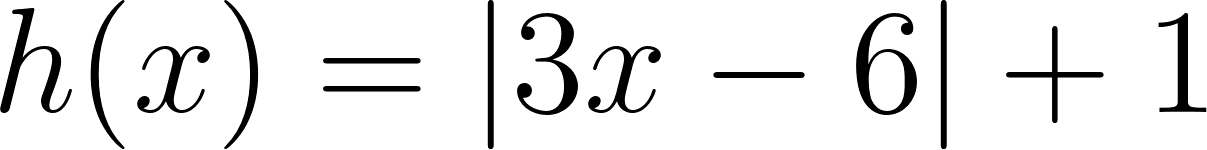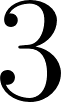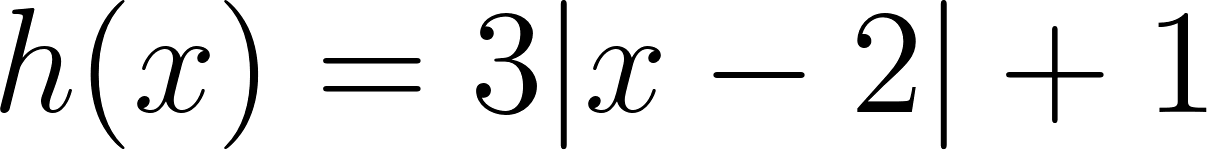×
Get Full Access to Calculus: Early Transcendentals - 1 Edition - Chapter 1.2 - Problem 38e
Get Full Access to Calculus: Early Transcendentals - 1 Edition - Chapter 1.2 - Problem 38e

×

# Shifting and scaling Use shifts and scalings | Ch 1.2 - 38EISBN: 9780321570567 2

## Solution for problem 38E Chapter 1.2

Calculus: Early Transcendentals | 1st Edition

• Textbook Solutions
• 2901 Step-by-step solutions solved by professors and subject experts
• Get 24/7 help from StudySoup virtual teaching assistantsCalculus: Early Transcendentals | 1st Edition

4 5 1 285 Reviews
27
1
Problem 38E

Shifting and Scaling:Use shifts and scalings to graph the given functions. Then check your work with a graphing utility. Be sure to identify an original function on which the shifts and scalings are performed.Step-by-Step Solution:

Step 1 of 4

Given function,By factoringout,

Rewrite asWe need shifts and scalings to graph the given functions.

Step 2 of 4

Step 3 of 4

##### ISBN: 9780321570567

Calculus: Early Transcendentals was written by and is associated to the ISBN: 9780321570567. Since the solution to 38E from 1.2 chapter was answered, more than 438 students have viewed the full step-by-step answer. This full solution covers the following key subjects: scalings, shifts, performed, given, graph. This expansive textbook survival guide covers 85 chapters, and 5218 solutions. This textbook survival guide was created for the textbook: Calculus: Early Transcendentals, edition: 1. The answer to “?Shifting and Scaling:Use shifts and scalings to graph the given functions. Then check your work with a graphing utility. Be sure to identify an original function on which the shifts and scalings are performed.” is broken down into a number of easy to follow steps, and 34 words. The full step-by-step solution to problem: 38E from chapter: 1.2 was answered by , our top Calculus solution expert on 03/03/17, 03:45PM.

Unlock Textbook Solution